GFG App
Open AppBrowser
Continue

Squares of the numbers are the values that are obtained by multiplying the number by itself. The behavior of Square and Square roots are opposite i.e; their definitions are Vice-Versa to each other. Hence, the square root when gets multiplied by itself, provides its original value. For Instance, the square of 3 is 9 while the square root of 9 is 3.

In a more generalized manner, we can say, if ‘P’ is the number given to us then, P2 represents the square of P. While the square root of P is expressed as √P. We can say that Square root is the Inverse Operation of Square.

## Squares

All square numbers can be expressed by a product of a number with itself.

4, 9, 25, 36, 49, 64, 81, 100 … and so on. Is there something special about these numbers? All these numbers are square numbers. To see this, let’s examine this pattern in these numbers

2*2 = 22 = 4

3*3 = 32 = 9

4*4 = 42 = 16

5*5 = 52 = 25

Mathematical Notation for a square: A square is represented with the number 2 represented as exponent on the number i.e. x2

25 = 52

16 = 42

9 = 3

These signs are saying “2 squared”, “3 squared” and “4 squared”. This notation is also called “superscript” or “power” of a number. Here, we have a power of 2, which is called the square of a number.

So in general, if a natural number p can be expressed as q2, where q is also a natural number, then p is a square number.

Question: Is 55 a square number?

Let’s look at the square of 7, 72 = 49 and square of 8, 82 = 64

55 lies between both of these squares, but there is no natural number between 7 and 8.

So, there is no natural number whose square is 55.

When it comes to addition, subtraction is the inverse operation of addition and vice versa. But in the case of a square, the square root is the inverse of square operation.

For example:

• 2 = 4, So the square root of 4 is 2.
• 3 = 9, So the square root of 9 is 3.

This can also be seen that (-3)2 and (3)2 both are equal to 9. So, 9 has two square roots.

So, we conclude that any positive square number has two square roots. But for the sake of simplicity, we will assume only the positive square root for now.

## Properties of Square Numbers

### Property 1: None of the square numbers end with 2, 3, 7, or 8 at the unit’s place

Let’s look at the table below, it contains the first twenty natural numbers and their squares.

If you study this table, you will realize that all the square numbers end with 1,4,9,6,0, and 5. None of these numbers end with 2,3,7 or 8 at units place.

But it’s not necessary that the numbers ending with 1,4,9,6,0 and 5 will be square numbers. For example: 46, it is not a square number.

### Property 2: Squares of even numbers are always even numbers and squares of odd numbers are always odd.

To support this statement, let’s take this example,

122 = 12 × 12 = 144. (both are even numbers)

112 = 11 × 11 = 121 (both are odd numbers)

302 = 30 × 30 = 900 (both are even numbers)

### Property 3: The number of zeros at the end of a perfect square is always even. In other words, a number ending in an odd number of zeros is never a perfect square.

2500 is a perfect square as the number of zeros is 2(even) and 25000 is not a perfect square as the number of zeros is 3 (odd).

## Square Roots

As we have already seen above, The Square root of a number is that value which when multiplied by itself will give its original number.

The square root is represented with the symbolcalled as radical or radix followed by the number which is called radicand i.e., √x.

For example:

√4 = 2

√9 = 3

√16 = 4

√25 = 5

## Properties of Square Root

### Property 1: If the units digit of a number is 2,3,7 or 8, then it does not have a root in N (the set of natural numbers).

132, 433, 688 does not have perfect square roots as unit digits are 2,3, and 8 respectively. This property is evident also from Property 1 of Square Numbers.

### Property 2: If a number ends in an odd number of zeros, then it does not have a square root. If a square number is followed by an even number of zeros, it has a square root in which the number of zeros, in the end, is half the number of zeros in the number.

2000 does not have a perfect square root as the number of zeroes is 3(odd). 900 have a perfect square root as the number of zeroes is 2(even). So square root of 900 will contain only 1 zero. (half of two zeroes).√900 = 30.

### Property 3: The square root of an even square number is even and that root of an odd square number is odd.

Example: √144 = 12 (both are even numbers) and √225 = 15 (both are odd numbers).

## Interesting Patterns in Square roots and Squares

Triangular numbers are the numbers whose dot patterns can be arranged as triangles.  For example: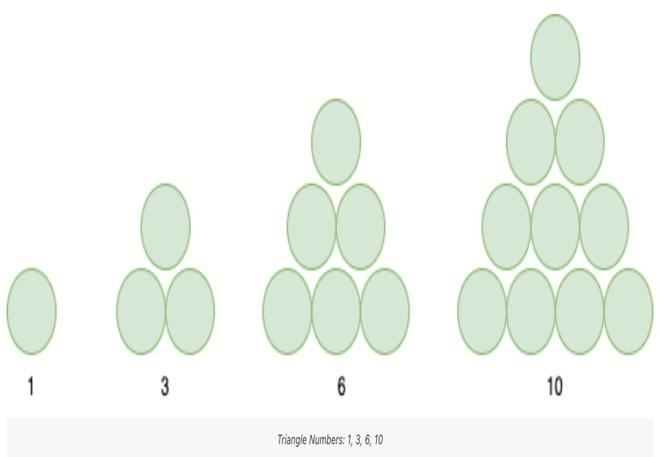If we combine two consecutive triangle numbers, we can get a number that is a square.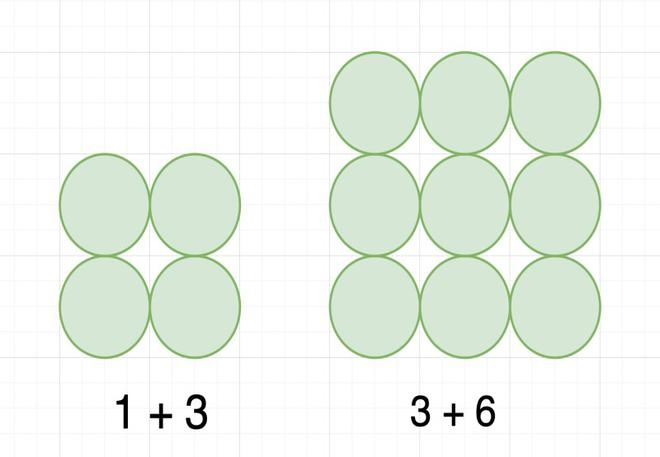### Squares of 1, 11, 111, 1111…

Squares of these numbers give rise to a beautiful pattern.

12                       =                        1

112                     =                    1  2  1

1112                   =               1  2  3  2  1

11112                 =          1  2  3  4  3  2  1

111112               =      1  2  3  4  5  4  3  2  1

### Squares of numbers with unit digit 5

Let’s assume a number with unit digit 5, i.e;

a52 = (10a + 5)2

= 10a(10a + 5) + 5(10a + 5)

= 100a + 50a + 50a + 25

= 100a (a + 1) + 25

Example:

252 = 625 = (2 × 3) hundreds + 25

352 = 1225 = (3 × 4) hundreds + 25

752 = 5625 = (7 × 8) hundreds + 25

1252 = 15625 = (12 × 13) hundreds + 25

## Sample problems on Squares and Square Roots

Finding Squares

Question 1: Find square of 23.

232 = (20 + 3)2

= 20(20 + 3) + 3(20 +3)

= 202 + 20 × 3 + 3 × 20 + 32

= 400 + 60 + 60 + 9

= 529

Question 2: Find the square of 39.

392 = (30 + 9)2

= 30 (30 + 9) + 9 (30 + 9)

= 302 + 30 × 9 + 9 × 30 + 92

= 900 + 270 + 270 + 81

= 1521

Finding Square Roots

We can find square roots through prime factorization of a number. Let’s look at some examples:

We can see that in the prime factorization of its square, each prime number occurs twice as compared to the prime factorization of the number itself.

By pairing the prime factors, we can get a square root.

Question 1: Find the square root of 144.

144 = (2 × 2) × (2 × 2) × (3 × 3)

= 22 × 22 × 32

= (2 × 2 × 3)2

= 122

Therefore, √144 = 12

Sometimes a number is not a perfect square.

Question 2: Is 2352 a perfect square? If not, find the smallest multiple of 2352 which is a perfect square. Find the square root of the new number.

2352 = 2 × 2 × 2 × 2 × 3 × 7 × 7

= 24 × 3 × 72

As the prime factor 3 has no pair, 2352 is not a perfect square.

If 3 gets a pair then the number will become perfect square.

2352 = 2 × 2 × 2 × 2 × 3 × 7 × 7

= 24 × 32 × 72

2352 = 22 × 3 × 7 = 84

### Finding the square root of decimal numbers

There are several methods of finding the square root of decimal numbers and the most famous and easiest method of manually finding the square root is called the Prime Factorization Method. For large numbers, we go for Long Division Method since the former method becomes tedious in the case of very large numbers.

Let’s learn how to find the square root of decimal numbers with an example:

Example: Square root of 19.36

Step 1: Make pairs of an integral part and decimal part of the number. Add a zero to the extreme right of the decimal part if required.Step 2: Find the perfect square of an integral part, find the number closest to the integral part (Either small or equal). In this case, the square of 4 is 16 which is closest to 19: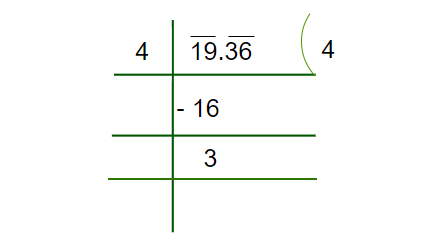Step 3: Put the decimal Part next to the Remainder obtained. Double the divisor of an Integral Part and place it in the next divisor, now we have to find the unit place value of this number.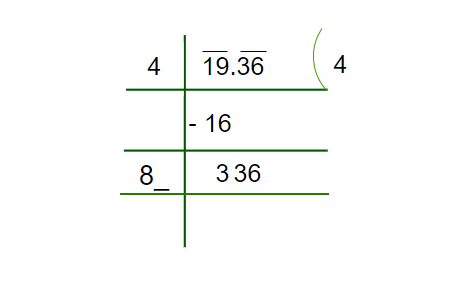Step 4: Now we have to find the unit place’s number which should be multiplied in order to get 336, here we can see, if we multiply 84 with 4, we will get 336.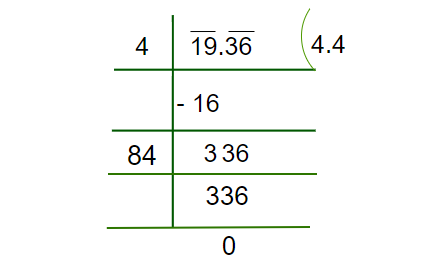Hence, we obtained 4.4 as the square root of 19.36

My Personal Notes arrow_drop_up